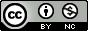# Scaling of Differential Equations

by Hans Petter Langtangen, Geir K. Pedersen### Subscribe to new books via dBooks.org telegram channel

Join
DescriptionDetailsHashtagsReport an issue

### Book Description

The book serves both as a reference for
various scaled models with corresponding dimensionless numbers, and as a
resource for learning the art of scaling.
A special feature of the book is the emphasis on how to create software
for scaled models, based on existing software for unscaled models.

Scaling (or non-dimensionalization) is a
mathematical technique that greatly simplifies the setting of input parameters in
numerical simulations. Moreover, scaling enhances the understanding of how
different physical processes interact in a differential equation model.
Compared to the existing literature, where the topic of scaling is frequently
encountered, but very often in only a brief and shallow setting, the present
book gives much more thorough explanations of how to reason about finding the
right scales. This process is highly problem dependent, and therefore the book
features a lot of worked examples, from very simple ODEs to systems of PDEs,
especially from fluid mechanics.

The text is easily accessible and
example-driven. The first part on ODEs fits even a lower undergraduate level,
while the most advanced multiphysics fluid mechanics examples target the
graduate level. The scientific literature is full of scaled models, but in most
of the cases, the scales are just stated without thorough mathematical
reasoning. This book explains how the scales are found mathematically.

This book will be a valuable read for anyone
doing numerical simulations based on ordinary or partial differential equations.

### Book Details

Title
Scaling of Differential Equations
Subject
Science and Mathematics
Publisher
Springer
Published
2016
Pages
149
Edition
1
Language
English
ISBN13
9783319327259
ISBN10
3319327259
ISBN13 Digital
9783319327266
ISBN10 Digital
3319327267
PDF Size
6.4 MB### Related BooksIn this book we discuss several numerical methods for solving ordinary differential equations. We emphasize the aspects that play an important role in practical problems. We conﬁne ourselves to ordinary differential equations with the exception of the last chapter in which we discuss the heat equation, a parabolic partial differential equation. T...This book presents a comprehensive survey of modern operator techniques for boundary value problems and spectral theory, employing abstract boundary mappings and Weyl functions. It includes self-contained treatments of the extension theory of symmetric operators and relations, spectral characterizations of selfadjoint operators in terms of the anal...Flow of ions through voltage gated channels can be represented theoretically using stochastic differential equations where the gating mechanism is represented by a Markov model. The flow through a channel can be manipulated using various drugs, and the effect of a given drug can be reflected by changing the Markov model. These lecture notes provide...This book presents computer programming as a key method for solving mathematical problems. This second edition of the well-received book has been extensively revised: All code is now written in Python version 3.6 (no longer version 2.7). In addition, the two first chapters of the previous edition have been extended and split up into five new chapte...Modeling and simulation are powerful tools for explaining the world, making predictions, designing things that work, and making them work better. Learning to use these tools can be difficult; this book is my attempt to make the experience as enjoyable and productive as possible. By reading this book - and working on the exercises - you will lear...This book presents computer programming as a key method for solving mathematical problems. There are two versions of the book, one for MATLAB and one for Python. The book was inspired by the Springer book TCSE 6: A Primer on Scientific Programming with Python (by Langtangen), but the style is more accessible and concise, in keeping with the needs o...